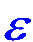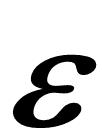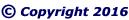"CLICK" below image; or, above blue ellipse to navigate.
 Emergent Ellipse Geometry Pythagorean Triangle Pairs (PTP)Construct a pairedPythagorean Triangle FGJin accordance with base, side, and hypotenuse as indicated in thebelow Table of Formulas. A pair of Pythagorean Triangleshave short sides,radius (r) and base (b),which vary bythe Elliptical Constant (EC) =.
 Construct a Pythagorean Triangle HIKin accordance with base, side, and hypotenuse as indicated in thebelow Table of Formulas. Pythagorean Triangles are triangleswith a right angle; and,all sides that are integers.= Elliptical Constant (EC) = One
 x = Integer (base 10)=b/2
 Table of Formulas
 Pythagorean Triangles
 FGJ = Pythagorean radius Triangle
 FG = wave (w)=b2/2–b = 2(x2–x)
 GJ = radius (r)=b–= 2x–FJ = hypotenuse (h)=b2/2–b+= 2x2–2x+HIK = Pythagorean Vector Triangle
 HI = base (b), V-wave, (Vw) = 2x
 HK = V-radius (Vr)=b2/4 –= x2–IK = V-hypotenuse (Vh)=b2/4+= x2+The Pythagorean Theorem, a2 + b2 = c2, is an an incredible coincidence of number theory, which arises from the Emergent Ellipsoid and the Natural source of Mathematics.

A Pythagorean Triangle Pair (PTP)
can be mapped to
every integer greater than One.

Four angles (two of the six angles are right angles)
and all six sides of a Pythagorean Triangle Pair
are of unlike values.

 "CLICK" top image; or, below blue ellipse to navigate.One must continuously ask: Why? Why? Why?; and, Why? again. And, realize that Fundamental Nature is the source of all Mathematics!
 Summary Epsilon equals One Proof of One Inverse Square Law Elliptical Constant Duality of Infinity
 Natural Function Brunardot Theorem revised Fibonacci Sequence Challenge to Academe Pulsoid Theorem Fundamental Intrinsic Time
 Salient Structural Parts Universal Locus Antimatter Heaven/God/Hell Philogic Entanglement

--- Page 2 ---